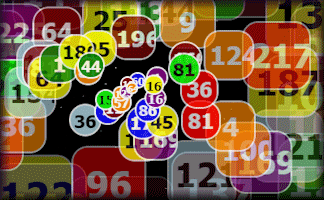### Each of the digits in the number ninety four is a square number. Let's call all numbers like that squigits!

1. Can you find a squigit which is divisible by 7 and larger than 50?

2. Which two squigits have a difference of 100?

3. Find a squigit whose square root is also a squigit.

4. Find a squigit that's also a prime number.

5. How many years last
century were squigits?

## A Mathematics Lesson Starter Of The Day

Share

Topics: Starter | Number

• S.Singh, Maltby Comprehensive, Rotherham
•
• Surely 0 is a square number so your answer for the years should also include 1900, 1901, 1904, 1909, 1910, 1940, 1990 making 16 in total.
• Steve Eastop, Margate, Kent.
•
• Although you can not form a square in reality from 0 items, (or a square of 0 by 0 items) nor can you form one from negative integers neither. For example, you can not draw a picture of a square containing say -9 points by -9 points (columns x widths). It is accepted, however, in Number Theory that the product of any two negative quantities always result in a POSITIVE AMOUNT! Therefore, one can 'square' a negative quantity (that doesn't exist in reality into a positive result that does)!
The first respondent was correct in that 0 SHOULD be included within the results/ solutions to these questions! The reason being that a SQUARE NUMBER is defined as: "A number that is the square of an integer: 1, 4, 9, 16, etc." Furthermore, an INTEGER is defined as: "The positive and negative whole numbers: 0, + or - 1, + or - 2, + or- 3,...." Hence 0 IS an integer and thus it CAN be squared as in the context of first definition of a square number given!
These defintions occur in the The Penguin book entitled: 'THE PENGUIN DICTIONARY OF MATHEMATICS' by John Daintith & R.D. Nelson! (Pages 175 and 306 respectively).

[Transum: Thanks for all of your comments. The answers below have now been altered to include zero as a square number.]
• Year 5, Primet Primary School
•
• The children at Primet Primary school would like to know if 0 is a square number. We have been having discussions and they believe if you have nought and multiply it by nought then you cannot make a square.
The children really enjoyed this challenge and found many different answers for each problem.
Thanks.

How did you use this starter? Can you suggest how teachers could present or develop this resource? Do you have any comments? It is always useful to receive feedback and helps make this free resource even more useful for Maths teachers anywhere in the world.

If you don't have the time to provide feedback we'd really appreciate it if you could give this page a score! We are constantly improving and adding to these starters so it would be really helpful to know which ones are most useful. Simply click on a button below:

Excellent, I would like to see more like this
Good, achieved the results I required
Satisfactory
Didn't really capture the interest of the students
Not for me! I wouldn't use this type of activity.

This starter has scored a mean of 3.0 out of 5 based on 383 votes.

Previous Day | This starter is for 29 June | Next Day

Your access to the majority of the Transum resources continues to be free but you can help support the continued growth of the website by doing your Amazon shopping using the links on this page. Below is an Amazon search box and some items chosen and recommended by Transum Mathematics to get you started.Teacher, do your students have access to computers?Do they have iPads or Laptops in Lessons? Whether your students each have a TabletPC, a Surface or a Mac, this activity lends itself to eLearning (Engaged Learning).Transum.org/go/?Start=June29

Here is the URL which will take them to a student activity about square numbers.

Transum.org/go/?to=SquareshootFor All: